# Variable Clustering 1 Variable Clustering Variable clustering finds

• Slides: 13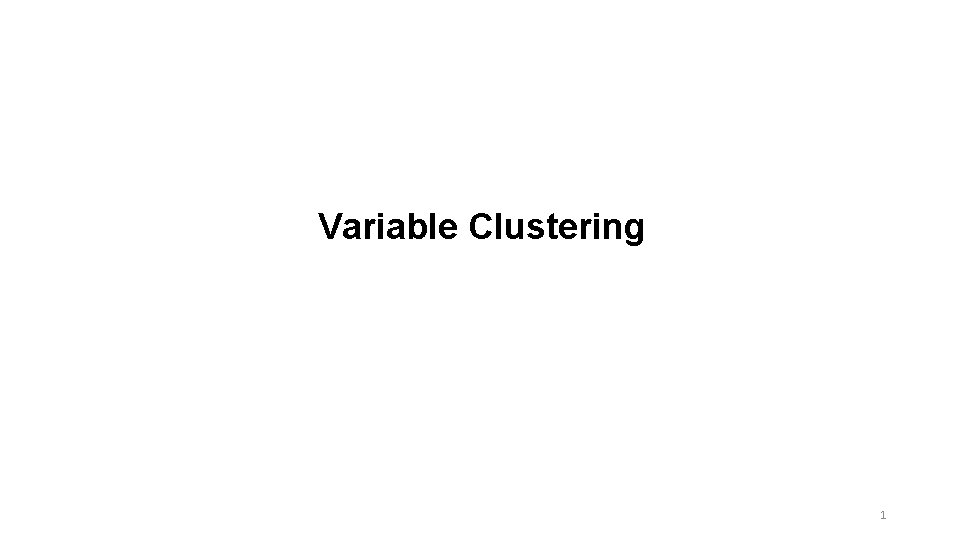Variable Clustering 1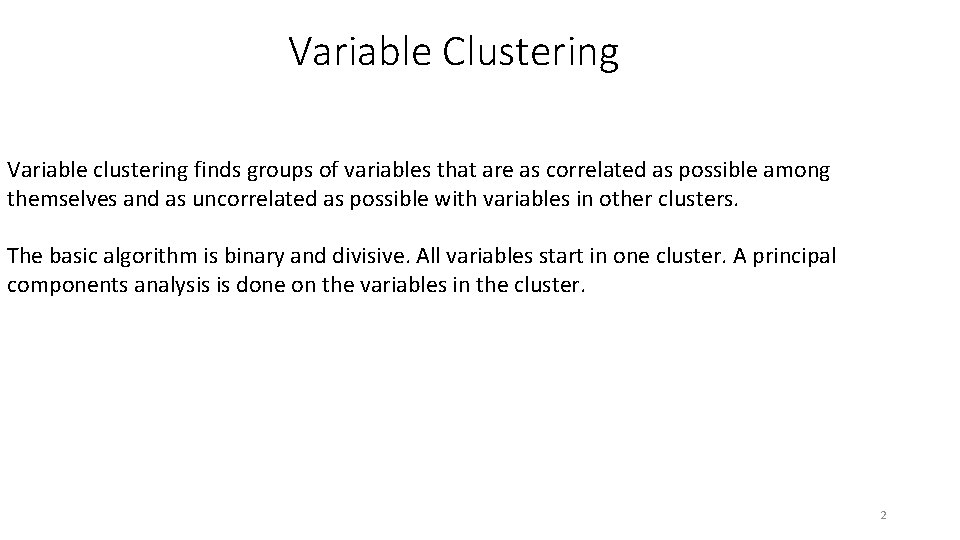Variable Clustering Variable clustering finds groups of variables that are as correlated as possible among themselves and as uncorrelated as possible with variables in other clusters. The basic algorithm is binary and divisive. All variables start in one cluster. A principal components analysis is done on the variables in the cluster. 2If the second eigenvalue is greater than a specified threshold (in other words, there is more than one dominant dimension), then the cluster is split. The PC scores are then rotated obliquely so that the variables can be split into two groups. This process is repeated for the two child clusters until the second eigenvalue drops below the threshold. 3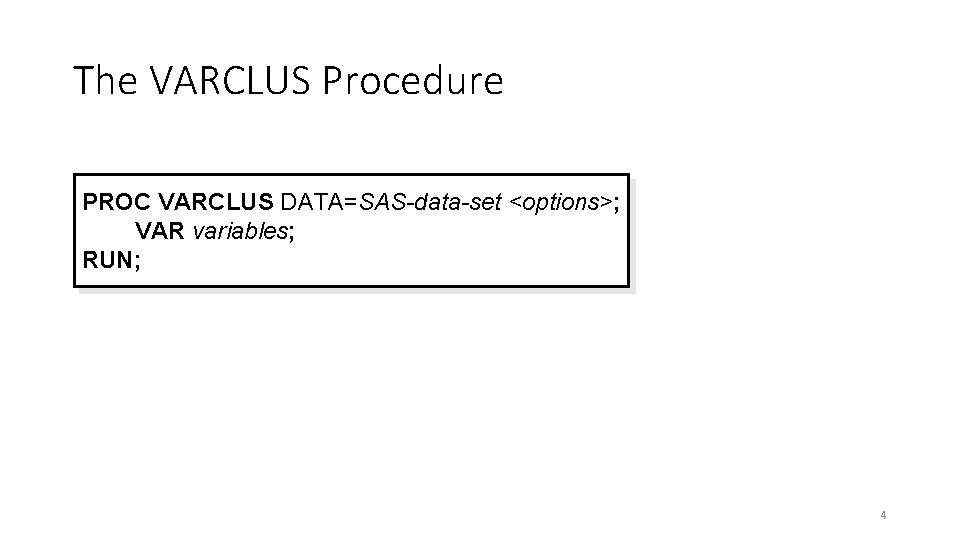The VARCLUS Procedure PROC VARCLUS DATA=SAS-data-set <options>; VAR variables; RUN; 4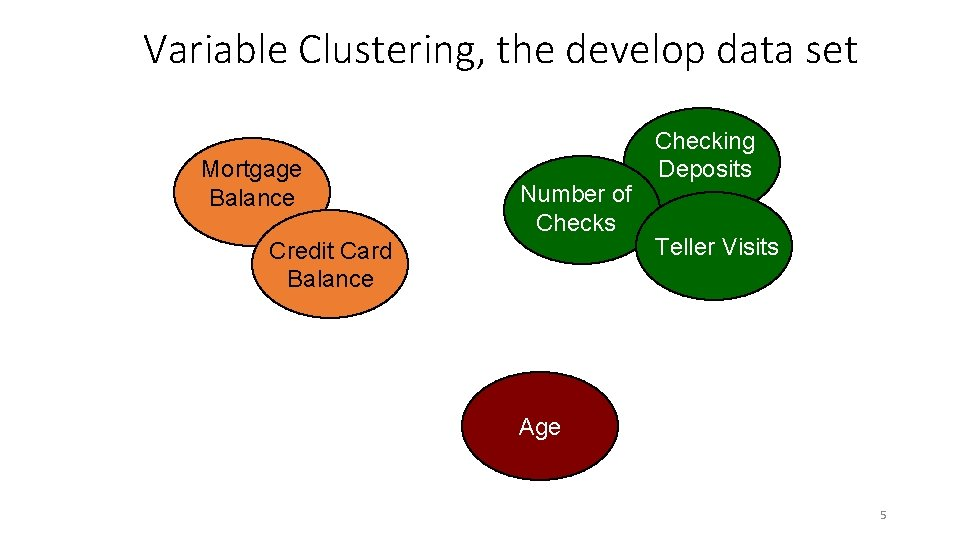Variable Clustering, the develop data set Mortgage Balance Number of Checks Credit Card Balance Checking Deposits Teller Visits Age 5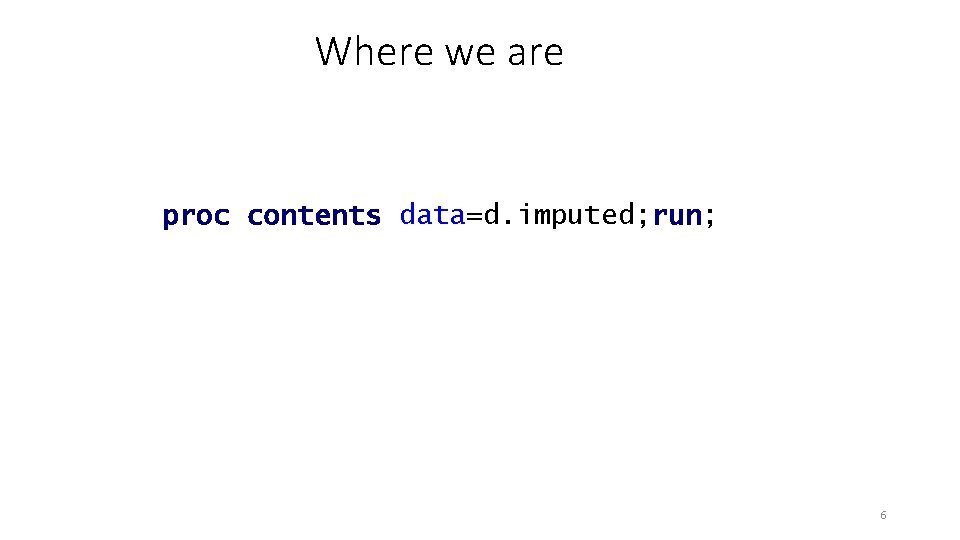Where we are proc contents data=d. imputed; run; 6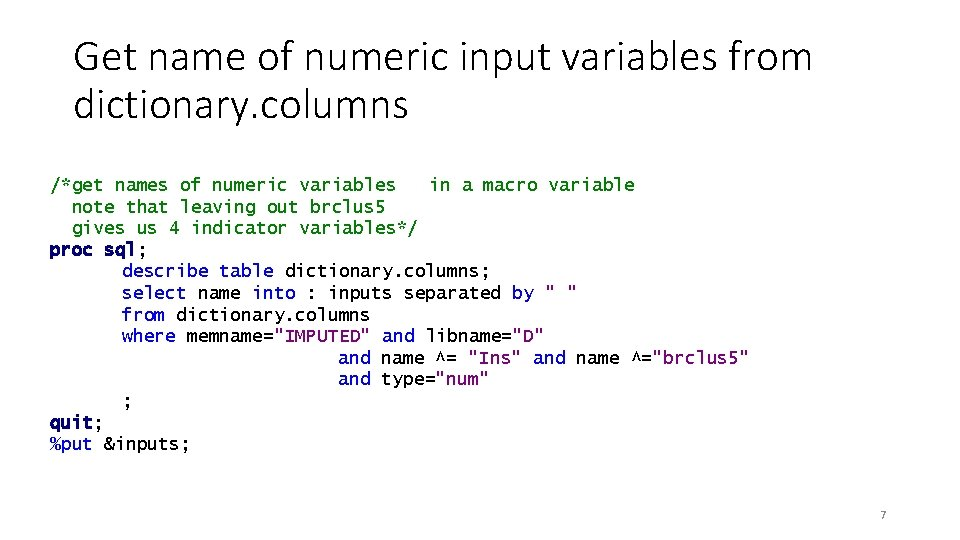Get name of numeric input variables from dictionary. columns /*get names of numeric variables in a macro variable note that leaving out brclus 5 gives us 4 indicator variables*/ proc sql; describe table dictionary. columns; select name into : inputs separated by " " from dictionary. columns where memname="IMPUTED" and libname="D" and name ^= "Ins" and name ^="brclus 5" and type="num" ; quit; %put &inputs; 7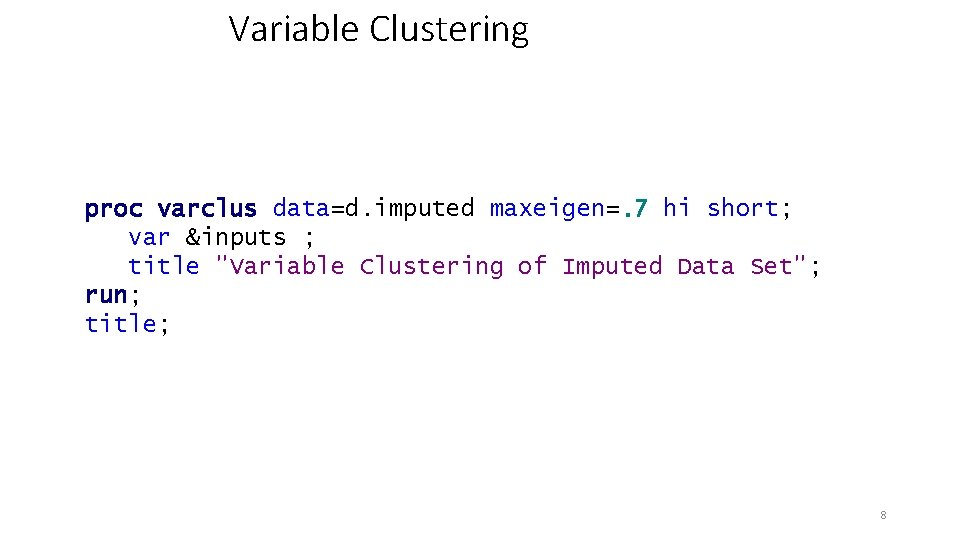Variable Clustering proc varclus data=d. imputed maxeigen=. 7 hi short; var &inputs ; title "Variable Clustering of Imputed Data Set"; run; title; 8Use ODS to get some stuff. ods output clusterquality=summary rsquare=clusters; proc varclus data=d. imputed maxeigen=. 7 short hi; var &inputs ; run; 9proc print data=summary; run; 10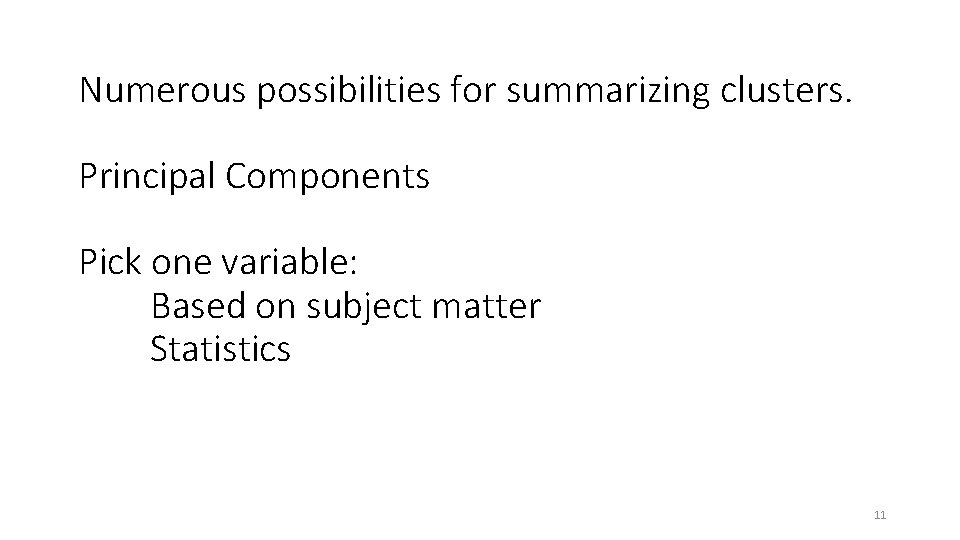Numerous possibilities for summarizing clusters. Principal Components Pick one variable: Based on subject matter Statistics 11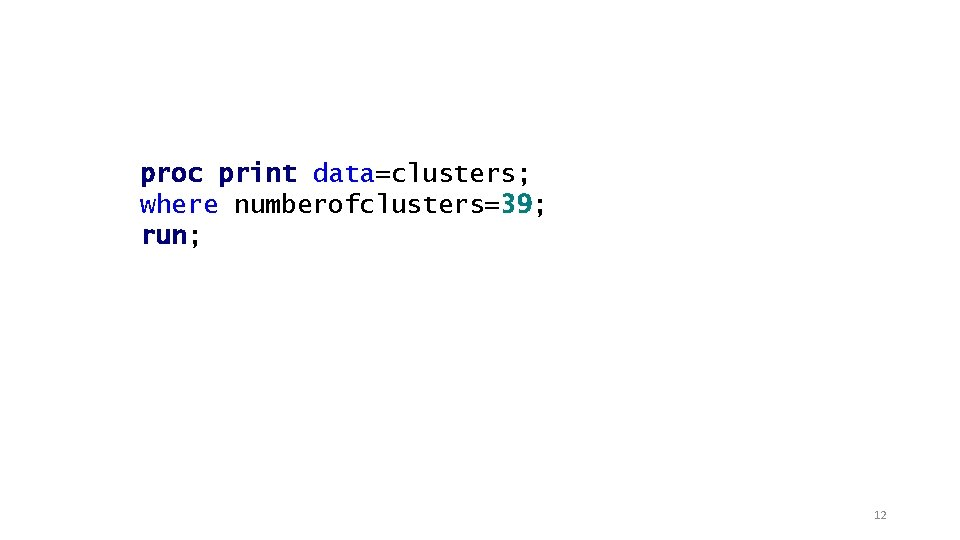proc print data=clusters; where numberofclusters=39; run; 12One variable per cluster /* Pick one variable per cluster for the first 10 The others are clusters of one variable */ %let reduced= MIPhone MICCBal Dep MM ILS MTGBal Income POS CD IRA brclus 1 Sav NSF Age Sav. Bal LOCBal NSFAmt Inv MIHMVal CRScore MIAcct. Ag Inv. Bal Dir. Dep CCPurc SDB Cash. Bk Acct. Age In. Area ATMAmt DDABal DDA brclus 2 CC HMOwn Dep. Amt Phone ATM LORes brclus 4; 13